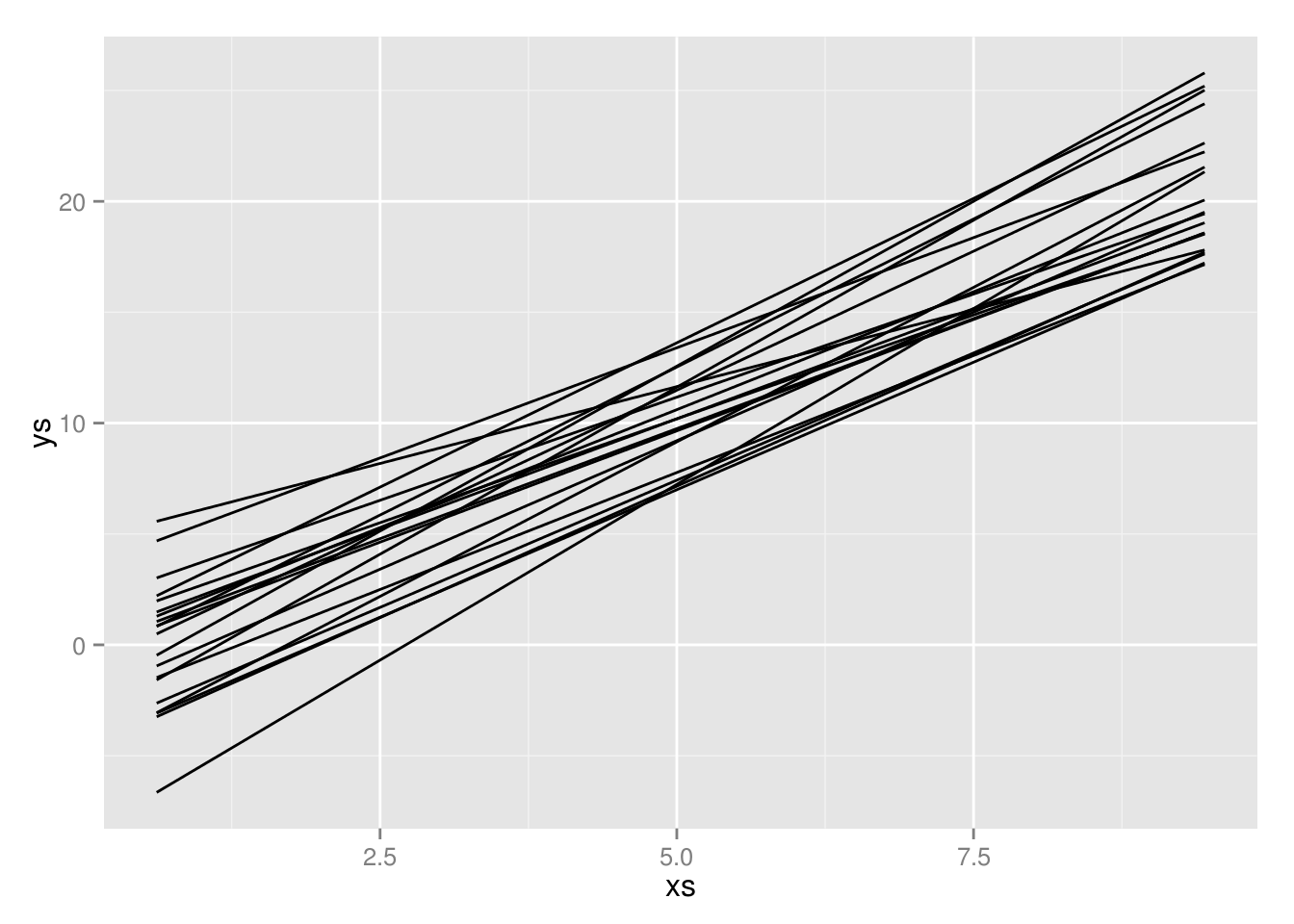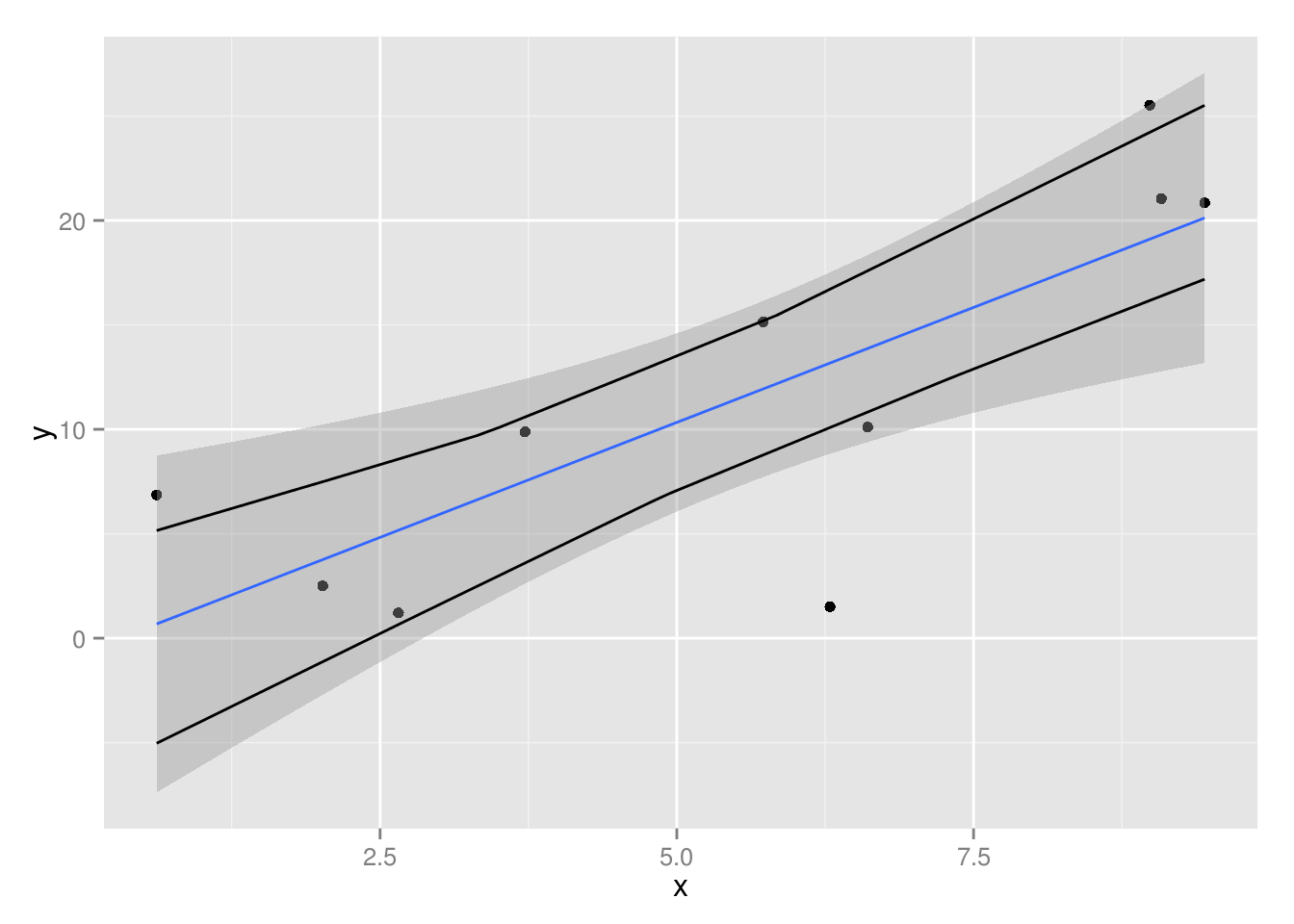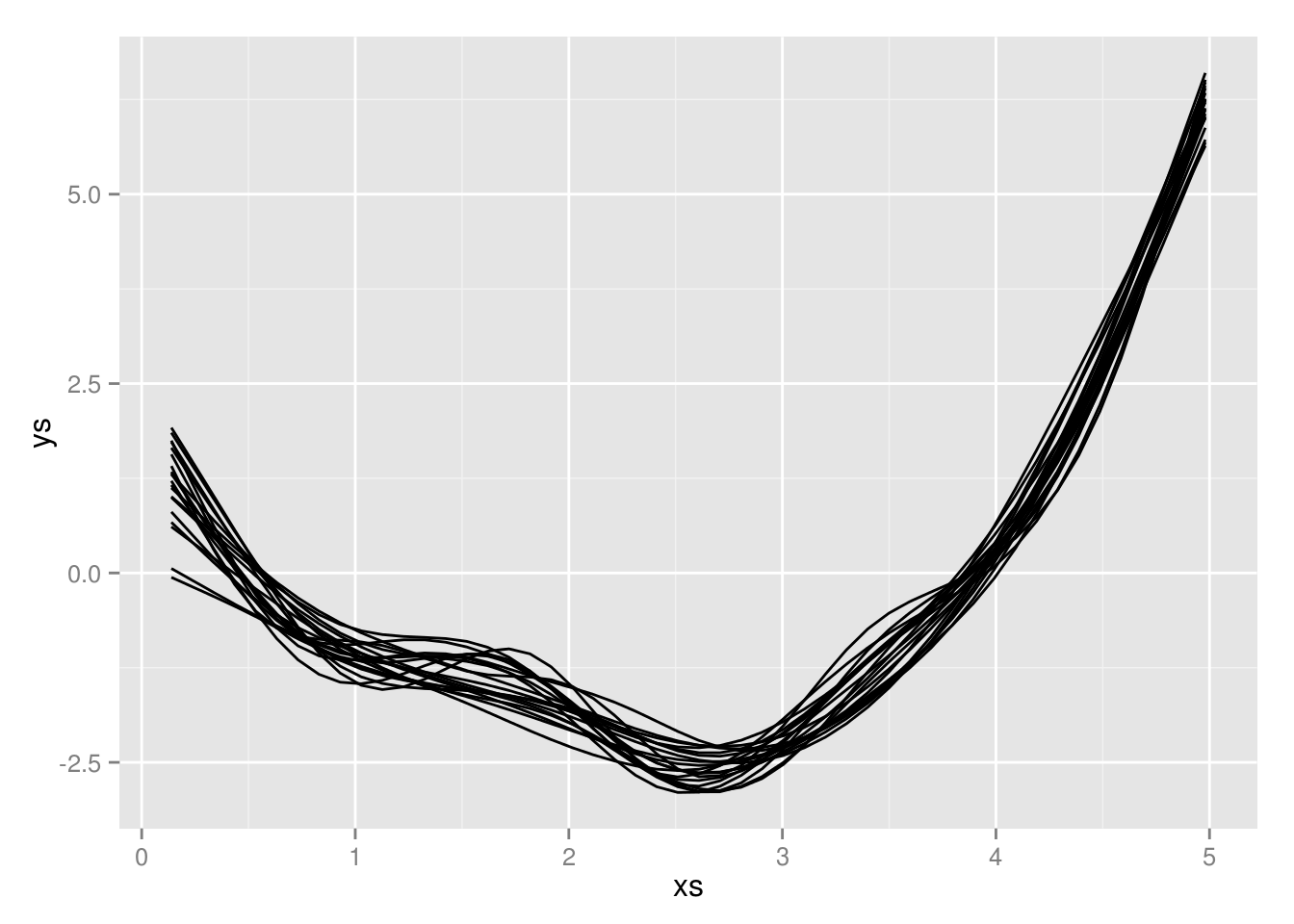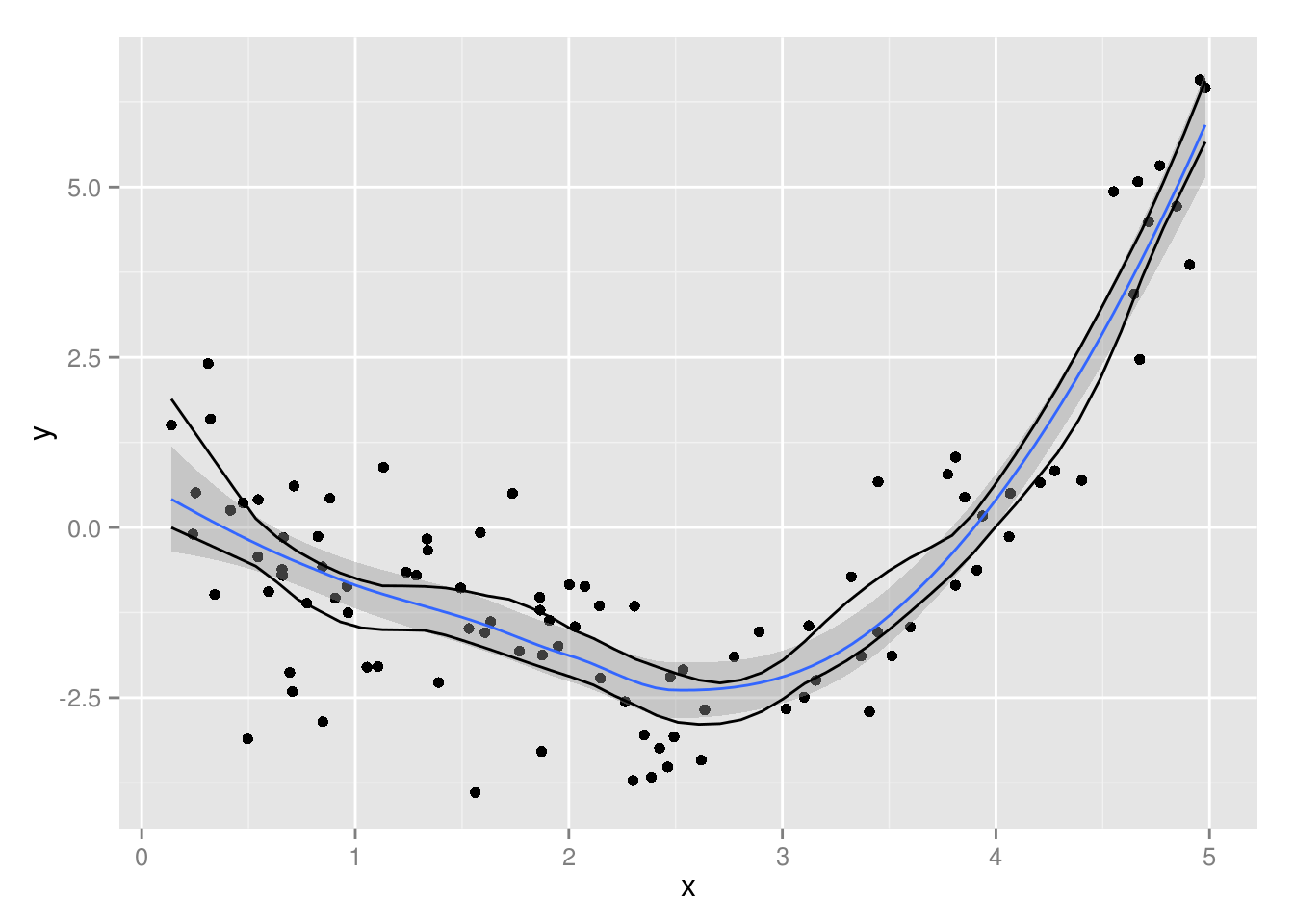Recently I was trying to put confidence intervals on a regression line, and I got some excellent advice from @davidjayharris on Twitter, who suggested the below method in an excellent gist. In the process of working with it, I ended up translating his method into dplyr syntax.

library(ggplot2)
library(dplyr)
library(magrittr)
library(mgcv)
set.seed(1)

df  <- data.frame(x = runif(10,min = 0,max = 10)) %>%
tbl_df %>%
mutate(y = x * 2 + rnorm(10, mean = 0,sd = 5))

sampled_models <- data.frame(nrep = seq_len(20)) %>%
group_by(nrep) %>%
do(df[df %>% nrow %>% sample.int(replace = TRUE),]) %>%
do(model = lm(y ~ x, data = .))

xs <- df$x %>% range %>% (function(rg) seq(rg,rg,length.out = 50)) boot_pred <- sampled_models %>% rowwise %>% do(data.frame(ys = predict(.$model,list(x = xs)))) %>%
ungroup %>%
cbind(xs)

boot_pred %>%
mutate(run = rep(1:20,each = 50)) %>%
ggplot(aes(x = xs, y = ys, group = run %>% factor)) + geom_line()boot_pred %>%
group_by(xs) %>%
summarize(up = quantile(ys, probs = 0.975),
lo = quantile(ys, probs = 0.025)) %>%
mutate(actual_data = lm(y ~ x, data = df) %>% predict(list(x = xs))) %>%
(function(d) {ggplot(df,aes(x = x, y = y)) + geom_point() + stat_smooth(method = "lm") +
geom_line(aes(x = xs, y = up),data = d) +
geom_line(aes(x = xs, y = lo),data = d)
})## a nonlinear function

very close to the original!

# Create fake data
x = runif(100, 0, 5)
y = .25 * x^3 - x^2 + rnorm(length(x))
data = data.frame(x = x, y = y)

sampled_models <- data.frame(nrep = seq_len(20)) %>%
group_by(nrep) %>%
do(data[data %>% nrow %>% sample.int(replace = TRUE),]) %>%
do(model = gam(y ~ s(x), data = .))

xs <- data$x %>% range %>% (function(rg) seq(rg,rg,length.out = 50)) boot_pred <- sampled_models %>% rowwise %>% do(data.frame(ys = predict(.$model,list(x = xs)))) %>%
ungroup %>%
cbind(xs)

boot_pred %>%
mutate(run = rep(1:20,each = 50)) %>%
ggplot(aes(x = xs, y = ys, group = run %>% factor)) + geom_line()boot_pred %>%
group_by(xs) %>%
summarize(up = quantile(ys, probs = 0.975),
lo = quantile(ys, probs = 0.025)) %>%
(function(d) {ggplot(data,aes(x = x, y = y)) + geom_point() + stat_smooth(method = "loess") +
geom_line(aes(x = xs, y = up),data = d) +
geom_line(aes(x = xs, y = lo),data = d)
})I feel like these operations could easily be made into a single function.